## how to seperate the two plots in proc sgplot with y2a? Can option= y2axis be used in proc template?

``````proc sgplot data=final;
/*discreteoffset to seperate the two box plot*/
/* the same offsets must be used in both YAXIS and Y2AXIS stmts */
scatter x=AVISITN y=mean  /  discreteoffset=0.5  YErrorUpper=uclm YErrorLower=lclm ;
series  x=AVISITN y=mean / ;

scatter x=AVISITN y=mean1  /Y2Axis discreteoffset=-0.5  YErrorUpper=uclm1 YErrorLower=lclm1;
series  x=AVISITN y=mean1/ Y2Axis;

/*reference line*/
refline 0  / axis=y;

/*grid : line */
yaxis   label="A (unit1)" offsetmax=0.1  offsetmax=0.1 values=(-2 to 0 by 0.2);
y2axis  label="A (unit2)" offsetmax=0.1 offsetmax=0.1 values=(-1.0 to 0 by 0.1);
run;``````

have :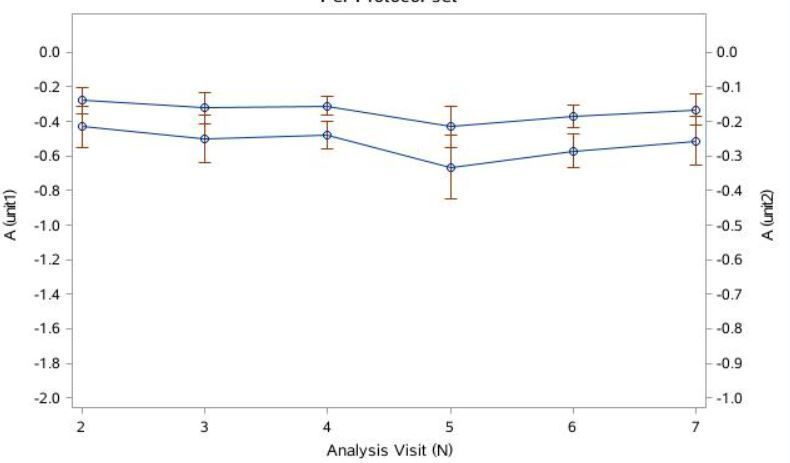dataset:

 Analysis _TYPE_ _FREQ_ lclm uclm mean lclm1 uclm1 mean1 Visit (N) 2 1 38 -0.55065 -0.31251 -0.43158 -0.1778 -0.10091 -0.13936 3 1 40 -0.63928 -0.36022 -0.49975 -0.20642 -0.11631 -0.16137 4 1 145 -0.55832 -0.3985 -0.47841 -0.18028 -0.12868 -0.15448 5 1 35 -0.84917 -0.48169 -0.66543 -0.2742 -0.15554 -0.21487 6 1 108 -0.66893 -0.47219 -0.57056 -0.216 -0.15247 -0.18423 7 1 71 -0.65607 -0.37041 -0.51324 -0.21185 -0.1196 -0.16573

want: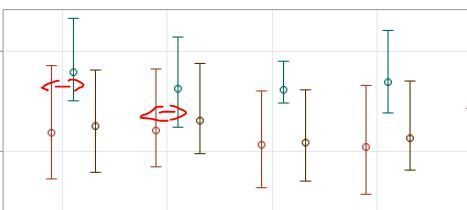use different axis for different units:

data want;
set have;
avisitn1=avisitn+0.1;
run;

1 ACCEPTED SOLUTION

Accepted SolutionsDanH_sas
SAS Super FREQ

## Re: how to seperate the two plots in proc sgplot with y2a? Can option= y2axis be used in proc templa

On the XAXIS statement add offsets to both ends:

XAXIS offsetmix=0.1 offsetmax=0.1;

That should be enough; bit if not, you can increase it.

8 REPLIES 8DanH_sas
SAS Super FREQ

## Re: how to seperate the two plots in proc sgplot with y2a? Can option= y2axis be used in proc templa

The key here is that the X axis variable you have is not discrete. Therefore, the DISCRETEOFFSET option is ignored. In this case, you need to explicitly tell the XAXIS to be discrete. Add the following line and your code should work as expected:

XAXIS type=discrete;

Hope this helps!
Dan

## Re: how to seperate the two plots in proc sgplot with y2a? Can option= y2axis be used in proc templa

hi, Dan, thanks. It works, but the graph is not imcomplete.

avisitn=avisitn+0.1 , which also works.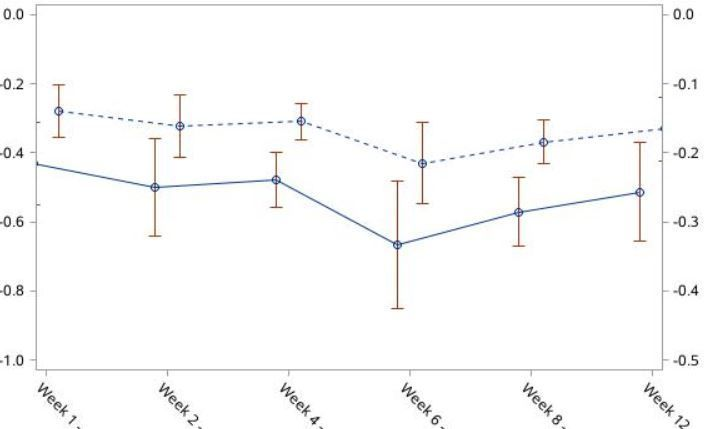DanH_sas
SAS Super FREQ

## Re: how to seperate the two plots in proc sgplot with y2a? Can option= y2axis be used in proc templa

On the XAXIS statement add offsets to both ends:

XAXIS offsetmix=0.1 offsetmax=0.1;

That should be enough; bit if not, you can increase it.

## Re: how to seperate the two plots in proc sgplot with y2? Can y2axis be used in proc template?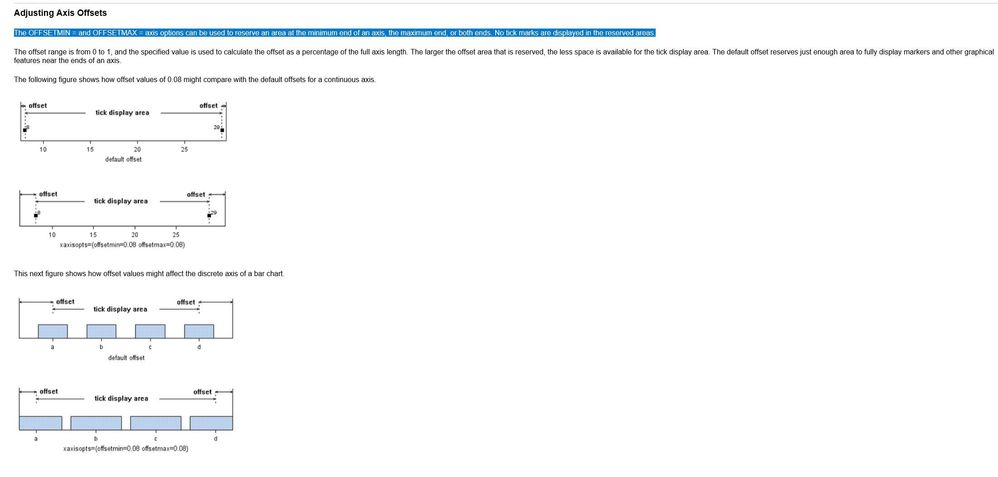https://support.sas.com/documentation/cdl/en/grstatgraph/63878/HTML/default/viewer.htm#n1tf9vsmrjtmv...

Dan, I appreciate your help, my figure looks good now.

Another question,  Can y2axis be used in proc template?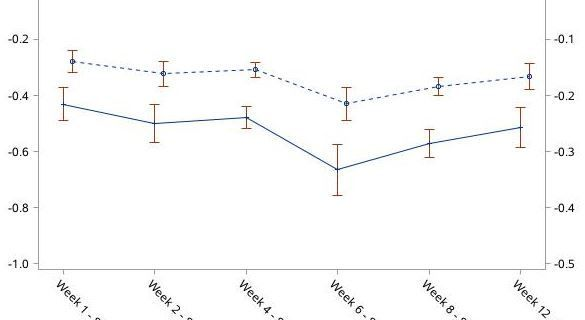DanH_sas
SAS Super FREQ

## Re: how to seperate the two plots in proc sgplot with y2? Can y2axis be used in proc template?

Yes, use the YAXIS=Y2 option on your SERIESPLOT.

## Re: how to seperate the two plots in proc sgplot with y2? Can y2axis be used in proc template?

Thanks again, Dan, I will try to use proc template for this figure

## Re: how to seperate the two plots in proc sgplot with y2a? Can option= y2axis be used in proc templa

I'm not sure I'm understanding what your looking for, but I just posted a question using offsetmin and offsetmax that shows how to completely separate the y2axis.  So the bottom of half of the plot shows data plotted against yaxis, and the top half shows data plotted against y2axis:

https://communities.sas.com/t5/Graphics-Programming/SGplot-with-3-y-axes/m-p/631567#M19592

(My question there is whether SGPLOT can do this with 3 y axes...)

Check out the Boston Area SAS Users Group (BASUG) video archives: https://www.basug.org/videos.

## Re: how to seperate the two plots in proc sgplot with y2a? Can option= y2axis be used in proc templa

Hi Quentin , my question has been solved,thanks.

I don't want show my figure with panelby.
Discussion stats
• 8 replies
• 519 views
• 5 likes
• 3 in conversation